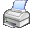﻿ bCell:GetColumnPercentage()

# bCell:GetColumnPercentage()Purpose

Determines the column percentage of the cell.

Class

bCell

Type

Method

Syntax

<oCell>:GetColumnPercentage(<iOptions>) Æ rPercentage

Arguments

 Options which should to be considered. The options below are supported:

 Option Description BCO_STARTCELL The percentage is determined for a start cell.   Notes: If the percentage is BCELL_PERCENTAGE_UNDEFINED, then the value BCELL_PERCENTAGE_NULL is used instead. BCO_ENDCELL The percentage is determined for an end cell.   Notes: If the percentage is BCELL_PERCENTAGE_UNDEFINED, then the value BCELL_PERCENTAGE_MAX is used instead.

 Data Type: DWord

Return Value

 rPercentage The determined column percentage.
 Data Type: Real8

Description

bCell:GetColumnPercentage() determines the column percentage of the cell.